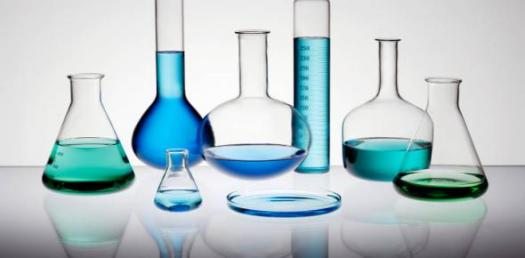10 Questions | Total Attempts: 153SettingsDid you know that everything is made out of chemicals? . Chemistry is a scientific discipline involved with elements and compound composed of atoms. Molecules and ions. Chemistry is the study of matter;it's composition, properties and reactivity. Try out this quiz to test your knowledge of chemistry.

• 1.
In a periodic table, elements are organized from left to right and from top to bottom. What is the name given to element moving from left to right?
• A.

Group

• B.

Period

• C.

Isotopes

• D.

Reactants

• 2.
What is the name given to elements moving from top to bottom?
• A.

Grouo

• B.

Period

• C.

Isotopes

• D.

Reactants

• 3.
• A.

Decreases from top to bottom down a group

• B.

Increases bottom to top up a group

• C.

Decreases right to left accros a group

• D.

Decreases left to right across a period

• 4.
Which of the following is a property of atom radius?
• A.

Decreases moving from right to left across a period

• B.

Increases moving from from bottom to top up a group

• C.

Increases moving from top to.bottom down a group

• D.

Increases moving from left to right accros a period

• 5.
What is ionization energy?
• A.

Energy needed for ironing

• B.

Amount of energy needed to remove an electron from anion in a gas state

• C.

Amount of energy needed to remove proton from an anion in a solid state

• D.

Amount of energy needes to remove neurons from an anion in a liquid state

• 6.
Which of th following is a property of ionization energy?
• A.

Increases moving from left to right across a period

• B.

Decreases moving from left to right across a period

• C.

All.of the above

• D.

Non of the above

• 7.
Which of the following is a feature of Ionization energy?
• A.

Increases moving from top to bottom down a group

• B.

Decreases moving from bottom to top up a group

• C.

Decreases moving from top to bottom down a group

• D.

Non of the above

• 8.
What is electron affinity?
• A.

Reflection of how readily an atom will accept an electron

• B.

Flexibility of cations

• C.

Ability of anions to become positively charged

• D.

Non of the above

• 9.
Which of the following is true about electronegativity?
• A.

The higher the electro negativity,the lower the attraction for bonding an electron

• B.

The higher the electro negativity, the lesser the beauty of the proton

• C.

The lower the electro negativity, the higher the attraction for bonding an electron

• D.

The higher the electro negativity, the higher the attraction for bonding an electron

• 10.
Which of the following is true?
• A.

Electro negativity increases down an element group

• B.

Electro negativity decreases across the period

• C.

Electro negativity decreases down the group

• D.

Non of the above

Related TopicsBack to top